Courses

# Test: Understanding Elementary Shapes - 3

## 20 Questions MCQ Test Mathematics (Maths) Class 6 | Test: Understanding Elementary Shapes - 3

Description
This mock test of Test: Understanding Elementary Shapes - 3 for Class 6 helps you for every Class 6 entrance exam. This contains 20 Multiple Choice Questions for Class 6 Test: Understanding Elementary Shapes - 3 (mcq) to study with solutions a complete question bank. The solved questions answers in this Test: Understanding Elementary Shapes - 3 quiz give you a good mix of easy questions and tough questions. Class 6 students definitely take this Test: Understanding Elementary Shapes - 3 exercise for a better result in the exam. You can find other Test: Understanding Elementary Shapes - 3 extra questions, long questions & short questions for Class 6 on EduRev as well by searching above.
QUESTION: 1

### How many centimetres make 3m?

Solution:

1m = 100 cm 3m = 3×100 cm = 300cm

QUESTION: 2

### How many metres is 805 cm?

Solution:

1m =100 cm
805cm=(800+5)cm=8m 5cm

QUESTION: 3

### If the string shown in the figure is pulled straight, then which one of the following will be the closest approximation of its length?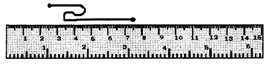Solution:

(4.5 - 2.5)+(4.5 - 3.0)+(7.5 - 3.5)=2+1.5

+ 4 = 7.5 cm = 8 cm (approx.)

QUESTION: 4

Which is the longest rectangle ?

Solution:

By observing & comparing the given figures, rectangle B is the longest rectangle.

QUESTION: 5

What is an angle which measures more than 0∘ and less than 90 called?

Solution:
QUESTION: 6

How is the measure of an angle expressed?

Solution:
QUESTION: 7

By what measure is the line m longer than the line n?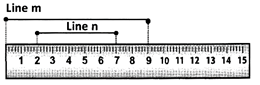Solution:
QUESTION: 8

An angle which measures 0 is called a/an ___ angle.

Solution:
QUESTION: 9

What type of an angle is ∠AOB?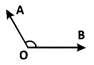Solution:
QUESTION: 10

What is an angle which measures 180 called?

Solution:

A straight angle measures180 .

QUESTION: 11

At 5:20 what type of angle is formed between the two hands of a clock?

Solution:
QUESTION: 12

l and m are two lines perpendicular to each other. What is the measure of the angle between them?

Solution:

The angle between perpendicular lines is 90°.

QUESTION: 13

179 is an example of which of these angles?

Solution:

179 90, 179 180  So it is obtuse.

QUESTION: 14

What is 89 an example of?

Solution:
QUESTION: 15

When an arm of an angle is extended then how does its measure change?

Solution:
QUESTION: 16

In ∠RST, what is the vertex ?

Solution:
QUESTION: 17

In ∠PRQ, which of the following are the two arms?

Solution:

⇒ In ∠PRQ, Q is a vertex of an angle.

⇒ RP and RQ are two rays of an angle.

⇒ So, RP and RQ​ are two arms of ∠PRQ.

QUESTION: 18

How do we write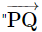is perpendicular to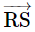'' symbolically?

Solution:
QUESTION: 19

What are the lines which lie on the same plane and do not intersect at any point?

Solution:
QUESTION: 20

How many pairs of parallel lines are there in the given figure?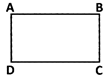Solution: